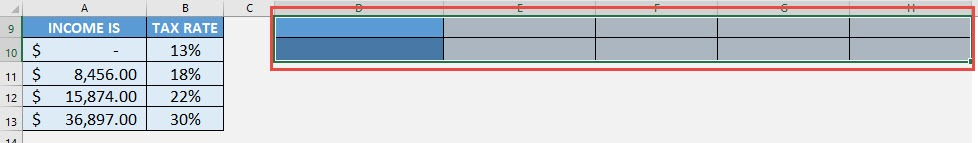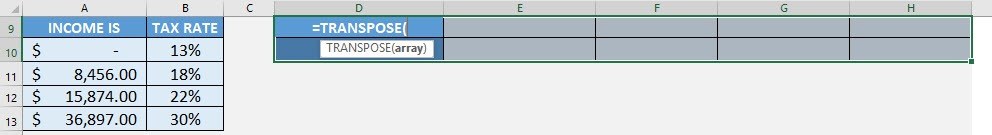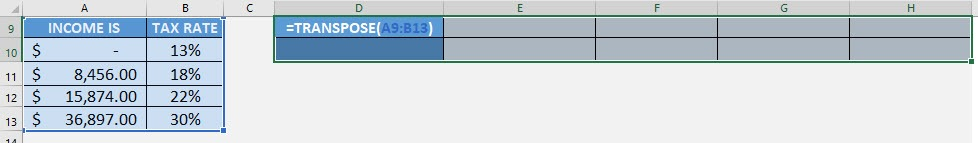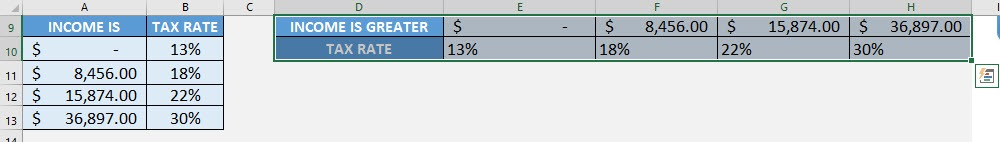What does it do?

Converts a vertical range of cells to horizontal, or vice versa

Formula breakdown:

=TRANSPOSE(array)

What it means:

=TRANSPOSE(the horizontal or vertical range of cells that you want to flip the orientation)

Let us say you have a horizontal table, and you want to flip it to become a vertical table, did you know that there is an Excel formula that can do that for you? That’s right, let us use the TRANSPOSE Formula!

Whenever you use this function, you should treat it as an array formula and it is very easy to do! You can use this to transform your horizontal table to a vertical table, or the other way around from a vertical table to a horizontal one.

I explain how you can do this below:STEP 1: Make sure to select your target table first with the same number of cells.

You can see we have selected a horizontal table that has the same number of cells (5 by 2) when compared to the original vertical table (2 by 5):While it is selected, we need to enter the TRANSPOSE function:

## =TRANSPOSE(STEP 2: The TRANSPOSE arguments:

## array

What is the range of cells that contains the data?

Select the cells containing the data that you want to flip the orientation:

## =TRANSPOSE(A9:B13)STEP 3: Once you finish typing the formula, ensure you press CTRL + SHIFT + ENTER for this to be treated as an array formula:Your table now has a horizontal orientation with just the TRANSPOSE Formula!

How to Use the Transpose Formula in Excel